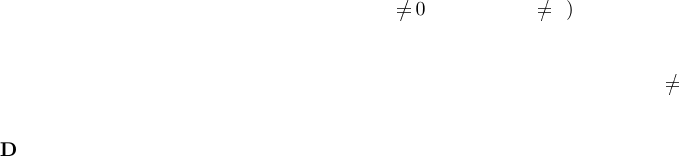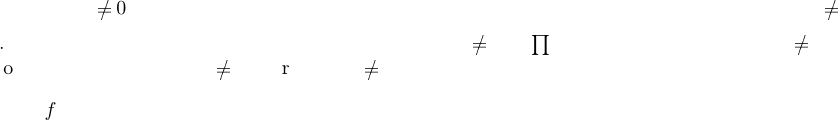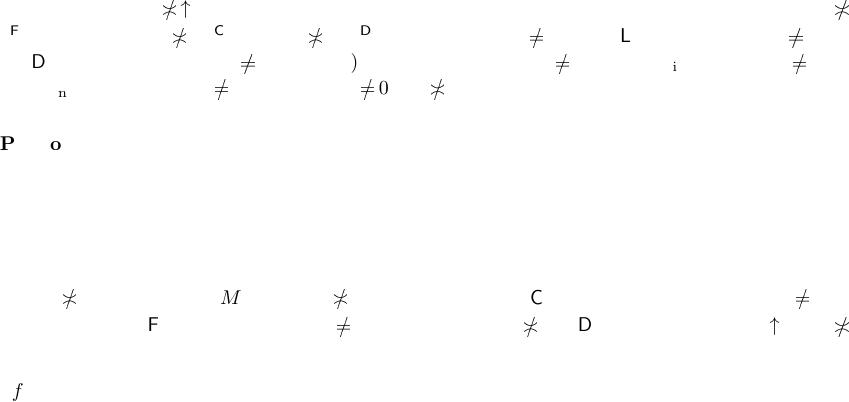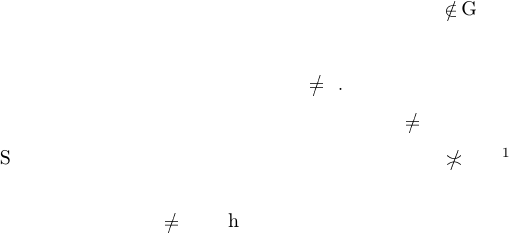Algebraic General Topology. Vol 1: Paperback / E-book || Axiomatic Theory of Formulas: Paperback / E-book

As an Amazon Associate I earn from qualifying purchases.Com pact funcoids
by Victor Porton
Email: porton@narod.ru
Web: http://www.mathematics21.org
Abstract
Compact funcoids are dened. Under certain conditions it’s proved that the reloid corre-
sponding to a compact funcoid is the neighbourdhood of the diagonal of the product funcoid.
Preface
This is a rough partial draf t. The proofs are with errors.
In order to understa nd it, rst read my boo k  and this draft article .
The rest
Deﬁnition 1. A funcoid f is directly compact iﬀ
∀F F: (hf iF
0 Cor hf iF
0).
Obvious 2. A funcoid f is d irectly compact iﬀ a atoms dom f : Co r hf ia
0.
Deﬁnition 3. A funcoid f is reversely compact iﬀ f
1
is directly compact .
Deﬁnition 4. A funcoid is compact iﬀ it is both directly compact and reversely compact.
Proposition 5.
Q
RLD
a =
RLD
Q
idom a
(
RLD
)
1
a
i
for every indexed family a of pri ncipal ﬁlters.
Proof. Because
Q
idom a
(
RLD
)
1
a
i
G R
Q
RLD
a.
[TODO: More detailed proof.]
Lemma 6.
Q
idom a
RLD
Cor a
i
= Cor
Q
RLD
a.
Proof. Cor
Q
RLD
a =
d
{↑
RLD
Q
A | A up a} =
RLD
T
{
Q
A | A up a} =
RLD
T
{
Q
A | A
P
Q
U, i dom a: A
i
up a
i
} =
RLD
T
{
Q T
K
i
| K PP
Q
U, i dom a: K
i
P up a
i
} =
RLD
T
{
Q
(
RLD
)
1
Cor a
i
| i dom a} =
RLD
Q
idom a
RLD
Cor a
i
.
[TODO: Check for little errors.]
Corollary 7.
Q
in
RLD
hCoCompl f
i
iX
i
=
D
CoCompl
Q
(A)
f
E
Q
RLD
X for e very n-indexed families
f of funcoid s and X of ﬁlters on the same set (wit h Src f
i
= Base(X
i
) for every i n).
1Proof.
Y
in
RLD
hCoCompl f
i
iX
i
=
Y
in
RLD
Cor hf
i
iX
i
=
Cor
Y
in
RLD
hf
i
i X
i
= (*)
Cor
Y
in
RLD
hf
i
i Pr
i
RLD
Y
RLD
X
!
=
Cor
*
Y
(A)
f
+
Y
RLD
X =
*
CoCompl
Y
(A)
f
+
Y
RLD
X .
(*) You should verify the special case when X
i
= 0
F
for some i.
Theorem 8. Let f be an indexed family of funcoids.
[TODO: Reverse theore m (for non -least
funcoids).]
1.
Q
f is directly co mpact if every f
i
is directly compact .
2.
Q
f is reversely compact if every f
i
is reversely compact.
3.
Q
f is compact if e very f
i
is compact.
Proof. It is enough to prove only the ﬁrst statement.
Let each f
i
is directly compact .
Let h
Q
f ia
0. Then h
Q
f ia =
D
Q
(A)
f
E
a =
Q
idom f
RLD
hf
i
iPr
i
RLD
a. Thus every hf
i
iPr
i
RLD
a
0. Consequently by compactness Cor hf
i
iPr
i
RLD
a
0;
Q
idom f
Cor hf
i
iPr
i
RLD
a
0;
Cor
Q
idom f
hf
i
iPr
i
RLD
a
0; Cor h
Q
f ia
0.
So
Q
f is directly compact.
I will denote the diagonal relation.
Proposition 9. The following expressions are pairwise equal:
1. hf × f i
;
2.
F
{hf × f ip | p atoms };
3.
F
{hf ix ×
RLD
hf ix | x F};
4. (RLD)
in
F
{hf ix ×
FCD
hf ix | x F};
5. (RLD)
in
F
{f (x ×
FCD
x) f
1
| x F};
6.
F
{((RLD)
in
f) (x ×
RLD
x) ((RLD)
in
f)
1
| x F}.
27. (RLD)
in
(f
1
f ) [TODO: Use this below.]
Proof. ??
Proposition 10. Le t g be a reloid and f = (FCD)g. Then hf × f i
g.
Proof. hf × f i
RLD
Y
RLD
[f × f]
RLD
Y
FCD
f ×
(C)
f
FCD
Y f
FCD
f
1
FCD
Y f f
1
FCD
Y f
FCD
Y f
FCD
Y
0 (RLD)
in
(f
FCD
Y )
0
(RLD)
in
f (RLD)
in
FCD
Y
0 (RLD)
in
f (RLD)
out
FCD
Y
0 (RLD)
in
f
RLD
Y
0
(RLD)
in
(FCD)g
RLD
Y
0 g
RLD
Y
0 g
RLD
Y .
Proposition 11. Le t f be a funcoid. Then V M V
1
GR hf × f i
M for every V GR f .
Proof. V M V
1
GR(f M f
1
) = GR
f ×
(C)
f
M GR hf × f i↑M = GR hf × f i
M.
[FIXME: Wrong direction of .]
Because
FCD
X
f ×
(C)
f
FCD
M
RLD
X
hf × f i↑
RLD
M (FCD)(
RLD
X hf × f i↑
RLD
M)
0
(FCD)
RLD
X (FCD)hf × f i↑
RLD
M
0 (FCD)
RLD
X
(FCD)hf × f i↑
RLD
M
FCD
X
(FCD)hf × f i↑
RLD
M;
f ×
(C)
f
FCD
M (FCD)hf × f i↑
RLD
M
GR
f ×
(C)
f
FCD
M GR (FCD)hf × f i↑
RLD
M GR hf × f i↑
RLD
M
Proposition 12. hf × f i
M g
RLD
M g
1
whenever (FCD)g = f for a reloid g.
Proof. For every V GR g we have V M V
1
GR hf × f i
M. Thus g
RLD
M g
1
=
d
{V M V
1
| V GR g }
d
GR hf × f i
M = G R hf × f i
M.
Corollary 13. hf × f i
M
f ×
(C)
f
M.
Corollary 14. V V
1
G R hf × f i
; f f
1
hf × f i
.
Proof. ??
Lemma 15. Cor hf × f i
g if (FCD)g = f where (FCD)g = f for a T
1
-separable reloid g.
Proof. ??
Remark 16. I attempted to generalize the below theorem more t han the standard g e ne ral topology
theorem about correspondence of compact and uniform spaces, but haven’t really succe eded much,
as it appears to be needed that the reloid in question is reﬂexive, symmetric, and transitive, that
is just a uniform space as in the st andard general topology.
Theorem 17. Let f be a T
1
-separable compact reﬂexive symmet ric funcoid and g be a reloid such
that
1. (FCD)g = f ;
2. g g
1
g.
3Then g = hf × f i
.
Proof. From the above hf × f i
g g
1
g.
[FIXME: Funcoids and reloids are confused.]
It’s remainded to prove g hf × f i
.
[FIXME: Possible er rors.]
Suppose there is U xyGR hf × f i
such that U
GR g.
Then {V \ U | V GR g} = g \ U would be a proper lter.
Thus by reﬂexivity hf × f i
(g \ U)
0.
By compactness of f × f, Cor hf × f i
(g \ U )
0.
Suppose ↑{(x; x)} hf × f i
(g \ U); then g \ U
hf
1
× f
1
i{(x; x)}; U hf
1
× f
1
i{(x;
x)} hf
1
× f
1
i what is impossible.
Thus there exist x
y such that {(x; y)} Cor hf × f i
(g \ U). Thus {(x; y)} hf × f i
g.
Thus by the lemma {(x; y)} w hat is impossible. So U GR g.
We have xyGR hf × f i
GR g; hf × f i
g.
Corollary 18. Let f is a T
1
-separable (the same as T
2
for symmetric transitive) compact funcoid
and g is a uniform space (reﬂexive, symm etric, and transitive endoreloid) such that (FCD)g = f.
Then g = hf × f i
.
An (incomplete) attempt to prove one more theorem follows:
Theorem 19. Le t µ and ν be uniform spaces, (FCD)µ be a compact funcoid. Then a map f i s a
continuous map from (FCD)µ to (FCD)ν iﬀ f is a (uniformly) continuou s map from µ to ν.
Proof. [FIXME: errors in this proof.]
We have µ = h(FCD)µ × (FCD)µi↑
RLD
f C
?
((FCD) µ; (FCD)ν). Then
f × f C
?
((FCD)(µ × µ); (FCD)(ν × ν))
(f × f) ( FCD)(µ × µ) (FCD)(ν × ν) (f × f )
For eve ry V GR(ν × ν) we have hg
1
iV h(FCD)(µ × µ)i{y} for some y.
hg
1
iV h(FCD)µ × (FCD)µi
RLD
= GR µ
hgihg
1
iV V
We need to prove f C(µ; ν) that is p GR ν q GR µ: hf iq p. But this follows from the
above.
Bib liography
 Victor Porton. Categorical product of funcoids. At http://www.mathematics21.org/binaries/product.pdf.
 Victor Porton. Algebraic General Topology. Volume 1. 2013.
4